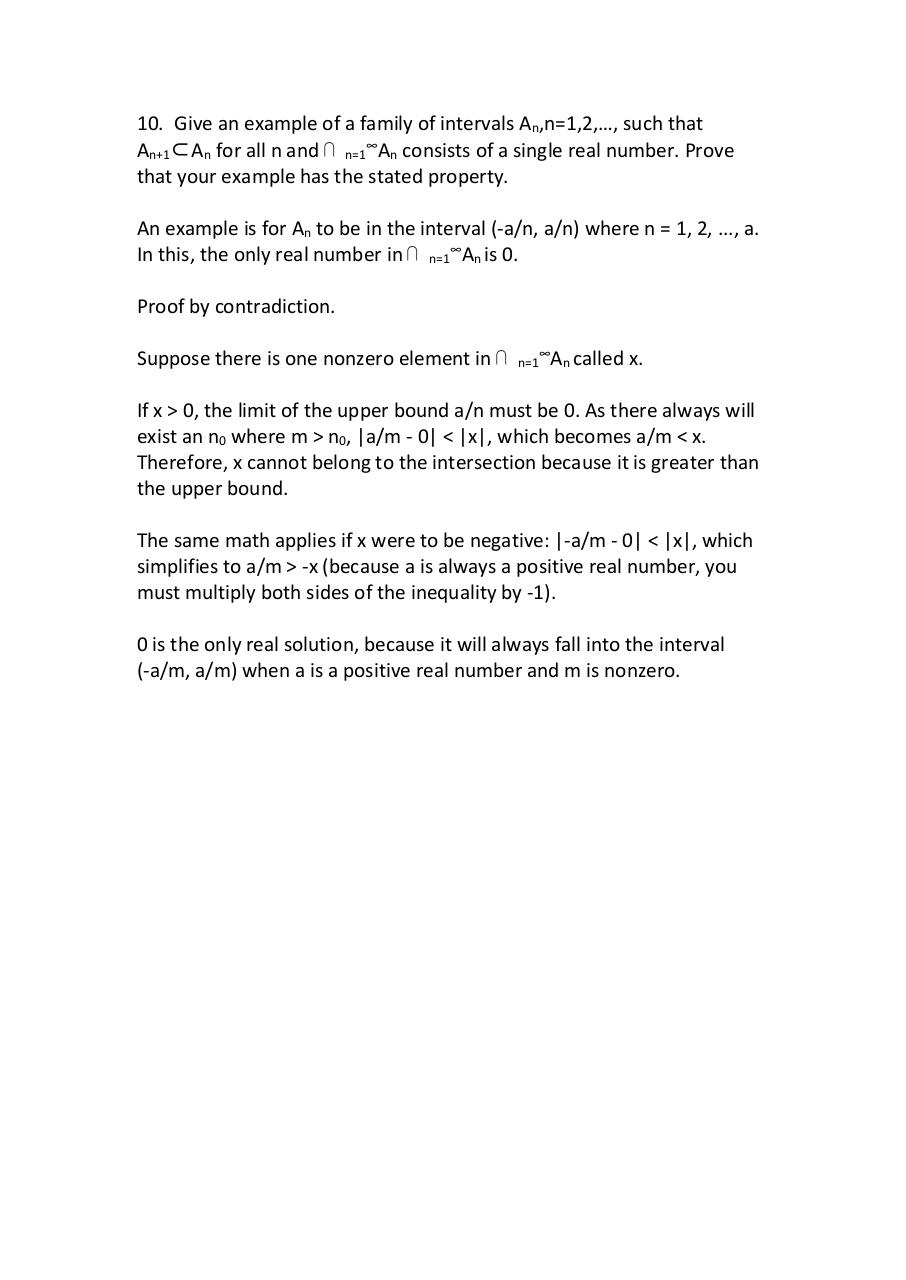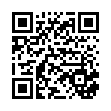# proof10 .pdf

### File information

Original filename: proof10.pdf
Author: vargh

This PDF 1.7 document has been generated by WPS Office / , and has been sent on pdf-archive.com on 28/08/2017 at 01:39, from IP address 108.218.x.x. The current document download page has been viewed 143 times.
File size: 73 KB (1 page).
Privacy: public file

proof10.pdf (PDF, 73 KB)

### Document preview

10. Give an example of a family of intervals An,n=1,2,…, such that
An+1⊂An for all n and ⋂ n=1∞An consists of a single real number. Prove
that your example has the stated property.
An example is for An to be in the interval (-a/n, a/n) where n = 1, 2, ..., a.
In this, the only real number in ⋂ n=1∞An is 0.
Suppose there is one nonzero element in ⋂

n=1

An called x.

If x &gt; 0, the limit of the upper bound a/n must be 0. As there always will
exist an n0 where m &gt; n0, |a/m - 0| &lt; |x|, which becomes a/m &lt; x.
Therefore, x cannot belong to the intersection because it is greater than
the upper bound.
The same math applies if x were to be negative: |-a/m - 0| &lt; |x|, which
simplifies to a/m &gt; -x (because a is always a positive real number, you
must multiply both sides of the inequality by -1).
0 is the only real solution, because it will always fall into the interval
(-a/m, a/m) when a is a positive real number and m is nonzero.#### HTML Code

Copy the following HTML code to share your document on a Website or Blog

#### QR Code### Related keywords